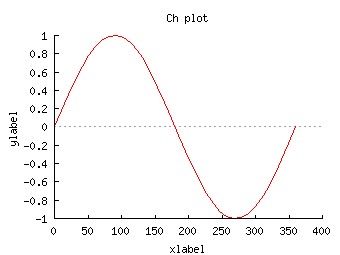SoftIntegration Demos
 Demos Ch Toolkit Demos Ch Mechanism Ch Control System Ch CGI Ch ODBC ChExcel C++ Library Demos Graphical Library Package Demos Ch NAG Statistics

SoftIntegration Graphical Library Demos.

An application program written using SoftIntegration Graphical Library (SIGL) can be compiled in C++. The same program, without any modification, can also run in Ch Professional Edition without compilation. For example, this program can be compiled in C++ with SIGL or readily executed in Ch.

#include <math.h> #include <chplot.h> #define NUM 36 int main() { double x[NUM], y[NUM]; class CPlot plot; int i; for(i=0; i<NUM; i++) { x[i] = i*360/(NUM-1); // assign x with values from 0 to 360 linearly y[i] = sin(x[i]*M_PI/180); } plot.title("Ch plot"); plot.label(PLOT_AXIS_X, "xlabel"); plot.label(PLOT_AXIS_Y, "ylabel"); plot.data2DCurve(x, y, NUM); plot.plotting(); } to display a plot with a sine wave shown below.Winplot is an example of how a plot can be displayed inside a window for an application. The interactive plotting can be accomplished in SIGL and Embedded Ch. The mathematical expressions entered by the user through a graphical user interface in Windows is processed by Embedded Ch and plotting is accomplished by SIGL. Winplotanim is an example of how to plot real-time data continuously.

There are over 100 demo programs in the distribution of SIGL. Click a plot below to view the corresponding program that generates the plot readily for display through the Web.